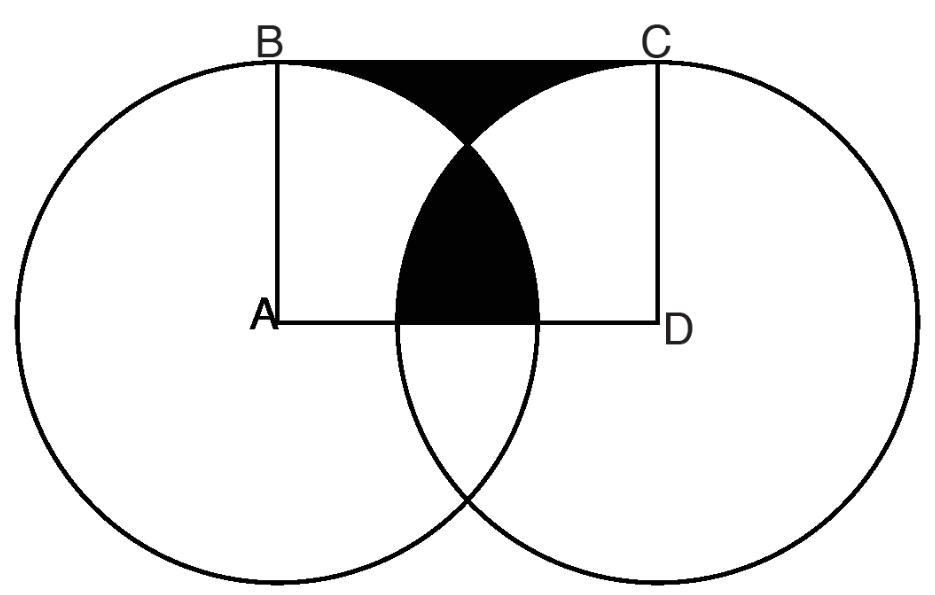# Circle problem

Geometry Level 3In the above diagram, $\overline{AB}$ and $\overline{DC}$ are radii of circles A and D with length 1. If ABCD is a rectangle, find its area.

Use the approximation $\pi = 3.14$ and answer to two decimal places.

Credit: New York Math League Contest

×